# “Why Compactness?”

Notes from the Margin, the twice-yearly CMS Studc publication featuring student writing for a general mathematical audience, has for several years provided a platform for topics from higher-order Fourier analysis to tenure-track job interviews. We are excited to present this first post on the Notes from the Margin blog, which will complement but not replace the semi-annual print publication. We invite submissions year-round at student-editor@cms.math.ca.

### “Why Compactness?”

#### Todd Schmid

With consequences such as the Upward Löwenheim-Skolem Theorem, the Compactness Theorem is one of the most foundational results in the study of first-order logic. It is common to see only a Henkin-style syntactic proof or a lay-it-on-the-anvil ultraproduct proof of first-order compactness. However illuminating these proofs are, neither of them illustrate a topological picture of what the theorem represents. As one might guess, this picture is where the theorem gained its name.

A few clarifications before we begin: We define an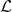theory to be a set of first-order logic sentences written in the languagewhich is closed under logical consequence. For example, the uniqueness of the group-theoretic inverse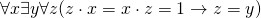is a sentence in the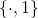-theory of groups, because it follows from the group axioms. An-theory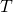is called consistent if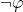does not belong towhen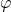belongs to. Note that I have chosen to draw no distinction between two sentences which are logically equivalent, and that “iff” should read “if and only if.”

Compactness Theorem. Fix a language of symbols, and letbe an-theory. Thenis consistent iff every finite subset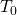ofis consistent.

Considering the compactness theorem is a statement about consistent-theories, it should seem sensible to topologize this collection. There turns out to be a natural way of doing this, but the fact of the matter is this: The set of all consistent-theories is unnecessarily complicated for our purposes! All we need to encode in our open sets are which finite subsets belong to an-theory. It is sufficient to focus our attention on a much better behaved collection of-theories.

Definition. An-theoryis complete if for any-sentence, either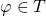or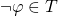.

In other words, complete theories decide on the truth-value of every sentence. To motivate the attention we give this special class of theories, one should verify for themselves the following properties of complete theories, omitting the parenthetical content either altogether or not at all:

(1) Each (consistent)-theory is contained in some complete (and consistent)-theory,

(2) any intersection of (consistent)-theories is also a (consistent)-theory, and

(3) an-theory is determined precisely by the set of complete-theories which contain it.

In particular, (3) holds for single sentences.

I will denote the collection of complete and consistent-theories with. We define a topology onas follows: For each-sentence, let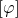denote the collection of-theories containing. Then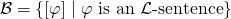is a basis for a topology called the Stone topology on(named after the mathematician M. H. Stone). To see that this is true, notice that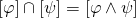, that, and that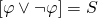. The theorem which follows is exactly the connection between topological and logical compactness.

Lemma. A subset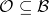coversiff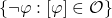is inconsistent.

Proof.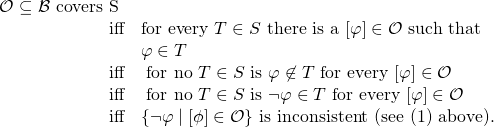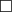Theorem.is compact iff the Compactness Theorem holds.

Proof.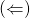Suppose that the Compactness Theorem holds, and let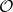be a collection from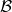which covers. Every element ofis of the form, so we can form a set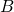out of the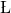-sentences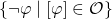. By the lemma,is an inconsistent set of sentences, so the Compactness Theorem tells us there is a finite subset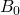ofwhich is inconsistent. We are left with a finite subset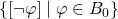ofwhich covers.was chosen arbitrarily, somust be compact.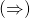Suppose thatis compact, and letbe an-theory. Ifis inconsistent, then the lemma tells us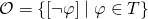is an open cover of.is compact, so a finite subset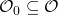covers. The collection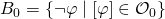is a finite subset ofwhich is inconsistent. On the other hand, ifhas a finite inconsistent subset, thenis inconsistent and there is nothing to show.What amazes me about the illustration above is that it approaches consistency in first-order logic from a purely topological perspective. Further inquiries in this direction can be found in  and , and a proof thatis compact can be found somewhere in .

Bibliography.

  A Topological Viewpoint of Logic and the Compactness Theorem (2016). T Schmid. Link.

 Ultrafilters, ultraproducts, and the Compactness Theorem (2009). A Caicedo. Link.

 Notes On Ultrafilters. A Kruckman. Link.Todd Schmid is an undergraduate at the University of Victoria. He likes long beach walks, the overlap of geometry and logic, and strange Scandinavian films. Among his favourite quotations is this one by César Vallejo: “Beloved be the ones who sit down.”